# Data Extraction using MATLAB

May 30, 2020

By  Aisik De

`lang: en_US`

## Introduction

Every day numerous facts are happening around us. We need them to predict our future and to improve our lifestyle. Effects like natural characteristics in geographic locations, the survey of the land, and geographic condition before building a dam or a power plant is an example. Maybe the result of a Lab experiment or Information Technology's encrypted data comes to the list. So, the collection of info in the database is done, but how can you obtain some crucial info that can turn the table? Something like the brand which has sold a maximum number of items in a particular region or the curve of active cases against the last two months of the time of noble Coronavirus Disease 2019, which need to flattened to survive the human race.

That is what is going to be presented in this blog by extracting.

1. Data extraction from statistics of COVID-19 excel sheet.

Now, due to the pandemic situation, Industry, Supply chain is suffering, the whole market is facing an economic breakdown. It becomes necessary to obtain the best selling brand to prioritize on them for cost reduction..
2. Data extraction from the sales chart of an imaginary region by MATLAB.

So, what has the situation of the whole world after COVID-19 pandemic become a threat to humankind, when surreal becomes real, and the universe is crying for it. How can we handle this situation, how to prevent the spread, and where to disinfect effectively?, We need the statistics of the Active, Recovered, and death statistics all over the world.Source: The Eastern Herald

We will process the data and get to know where is the death toll is most and what is the curve of active cases with time where the disinfection process should go on.Source: News Chief

Sales details are what we are going to focus on where the brand has the maximum number of sales in a particular region. With this data, we can plot the sales chart as well as the next quarter schedule, and a business plan can be developed. Customer demand in different regions can be analyzed, and further can be proved beneficial for the seller.

## Data for analysis

### Plotting of COVID-19 statistics

Theory:-

The sheet presents with DateRep, cases, deaths, Countries, GeoID, year, month, population.

We are going to extract only date, cases, and deaths and our task is

1. Store the daily cases of each country in data_cases. Store the daily death of each country in data_deaths.
2. Store the unique data of which statistics of every country is given.

The first step is to open the excel sheet in MATLAB and generate a function.Next, to the code, when, function is generated , below code will be automatically generated in MATLAB script.

### Code for Plotting of COVID-19 statistics

%%%%%%%%%%%%%%%%%%%%%%%%%%%%%%%%%%%%%

function tableout = importfile()
startRow = 2;
endRow = 13419;
workbookFile='COVID-19-geographic-disbtribution-worldwide.xlsx';
sheetName='COVID-19-geographic-disbtributi';
%% Import the data, extracting spreadsheet dates in Excel serial date format
for block=2:length(startRow)
raw = [raw;tmpRawBlock]; %#ok<AGROW>
dates = [dates;tmpDateNumBlock]; %#ok<AGROW>
end
raw(cellfun(@(x) ~isempty(x) && isnumeric(x) && isnan(x),raw)) = {''};
stringVectors = string(raw(:,[7,8,9,11]));
stringVectors(ismissing(stringVectors)) = '';
raw = raw(:,[2,3,4,5,6,10]);
dates = dates(:,1);
%% Replace non-numeric cells with NaN
R = cellfun(@(x) ~isnumeric(x) && ~islogical(x),raw); % Find non-numeric cells
raw(R) = {NaN}; % Replace non-numeric cells
R = cellfun(@(x) ~isnumeric(x) && ~islogical(x),dates); % Find non-numeric cells
dates(R) = {NaN}; % Replace non-numeric Excel dates with NaN
%% Create an output variable
I = cellfun(@(x) ischar(x), raw);
raw(I) = {NaN};
data = reshape([raw{:}],size(raw));
%% Create table
tableout = table;
%% Allocate imported array to column variable names
dates(~cellfun(@(x) isnumeric(x) || islogical(x), dates)) = {NaN};
tableout.dateRep = datetime([dates{:,1}].', 'ConvertFrom', 'Excel');
tableout.day = data(:,1);
tableout.month = data(:,2);
tableout.year = data(:,3);
tableout.cases = data(:,4);
tableout.deaths = data(:,5);
tableout.countriesAndTerritories = categorical(stringVectors(:,1));
tableout.geoId = categorical(stringVectors(:,2));
tableout.countryterritoryCode = categorical(stringVectors(:,3));
tableout.popData2018 = data(:,6);
tableout.continentExp = categorical(stringVectors(:,4));
% For code requiring serial dates (datenum) instead of datetime, uncomment
% the following line(s) below to return the imported dates as datenum(s).
% tableout.dateRep=datenum(tableout.dateRep);

Here the following points are to be noted:

1. workbookFile=’name.xlsx' startRow =2, %2 because the first usually be the heading of the columns.
2. endRow=the maximum number of row, or if 2018 or later is used, [val,~]=readtable(workbookFile)

can be used.

• The rest of the document, no change is required.

Our primary focus is on declaring the workbookFile, startRow, and endRow.

Next is the script, where the data will be processed and analyzed and plotted.

%%%%%%%%%%%%%%%%%%%%%%%%%%%%%%%%%%%%%

To process the data

%  Author: Aisik De
% Topic: Plotting statistics of COVID-19 in MATLAB
% Company: MATLABHelper
% Website: https://MATLABHelper.com
% Date : 22-05-2020
% With the help of this script we will extract data from excel and plot different cases of COVID-19

clc;

clear all;

close all;

%% data segregation

UniqueCountries=categories(Countries);

number_of_countries=length(UniqueCountries);

%%data fetching

% for loop used to segregate the cases and deaths.

for i=1:number_of_countries

data_cases{i}=data_specific_country.cases;

data_deaths{i}=data_specific_country.deaths;

data_date{i}=unique(data_specific_country.dateRep);

end

%%data plotting

%subplot is used to plot 1. date vs cases 2. date vs deaths

subplot(211);

plot(data_date{1},data_cases{1},'b',data_date{1}, data_deaths{1},'r');

legend('Confirmed cases','Deaths');

subplot(212);

plot(data_date{2},data_cases{2},'b',data_date{2}, data_deaths{2},'r');

legend('Confirmed cases','Deaths');

Here data is being processed and stored in 3 different arrays as discussed before, and plotting is done for two countries. No need to get confused a little thorough study and attention will get you straight. Grab a soft drink and chill out and then come down to the next section to follow the steps in MATLAB.

Following points are to be noted:

1. All the countries are stored in Countries variable. The unique countries are saved in UniqueCountries by using the categories command.
2. (Countries==UniqueCountries {i},:) gives the index of all the countries that are matching with the unique countries.
3. Basically we are extracting the data and storing it in
• data_cases
• data_deaths
• data_date
• And are plotted between data_date{1} vs data_cases{1}  and data_date{2},data_cases{2} using plot command.
• The legend is used to name the plot axis.

### Result of Plotting of COVID-19 statistics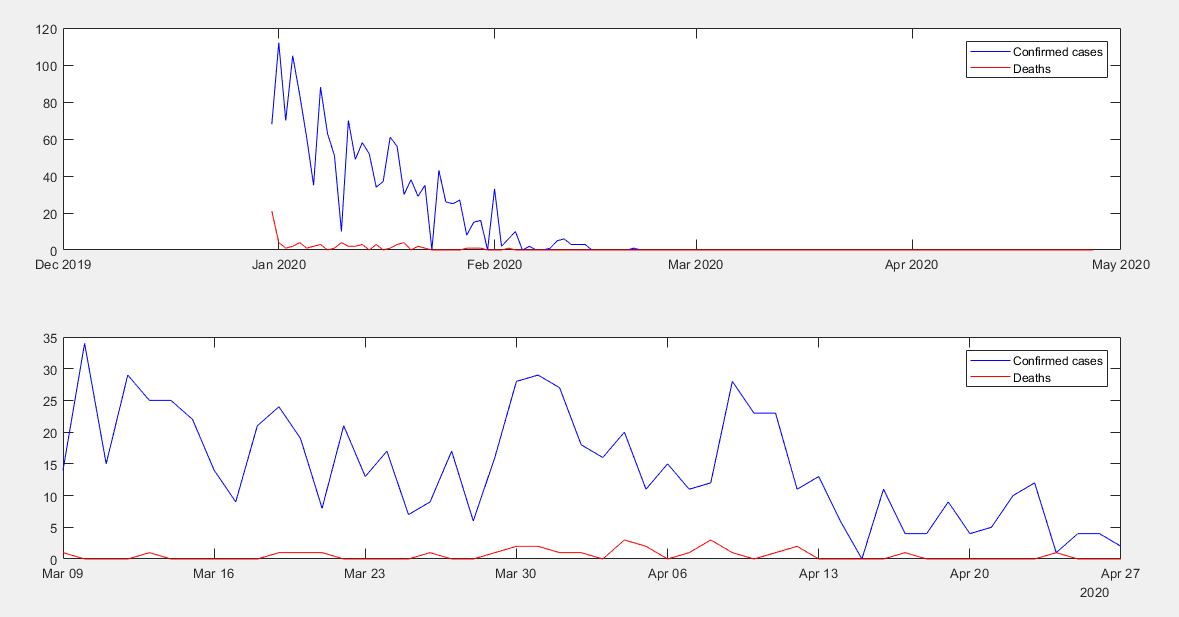Here the blue indicates the cases, and red represents death for Afghanistan and Albania.

The date is on the x-axis, and the data is on the y-axis.

## Determining the best selling brand

Theory:-

So, we get to know different statistics and results of COVID-19. Global lock-down is going on. Therefore now comes the role of a company(Imaginary) to determine the best selling brand to concentrate on those and neglect the other, which has barely any effect in the market, so here is what we do.

We have taken an example of Sales review or sales record of different brand in Central, East, and West Region went off in the past quarter. Now, the time has come to judge which brand has grabbed the most sales order in a region. It will be crowned as the 'Most Valuable Company’ and will be handled with primary focus.

The excel sheet can be downloaded from the Data section, second point.

The information contains order date, rep (brand), item in 3 different Region, number of units, and unit cost, and total price.

Our goal is to get the brand that has the most sell in each region.

### Code for Determining the best selling brand

%%%%%%%%%%%%%%%%%%%%%%%%%%%%%%%%%%%%%

function SampleData = sales()

%% Input handling

workbookFile="SampleData";

sheetName="SalesOrders";

dataLines='';

[row,~]=size(t)

%% Setup the Import Options and import the data

% Specify sheet and range

opts.Sheet = sheetName;

opts.DataRange = sprintf('A2:G%d',row+1);

% Specify column names and types

opts.PreserveVariableNames = true;

opts.VariableNames = ["OrderDate", "Region", "Rep", "Item", "Units", "Unit Cost", "Total"];

opts.VariableTypes = ["datetime", "categorical", "categorical", "categorical", "double", "double", "double"];

% Specify variable properties

opts = setvaropts(opts, ["Region", "Rep", "Item"], "EmptyFieldRule", "auto");

opts = setvaropts(opts, "OrderDate", "InputFormat", "");

% Import the data

SampleData = readtable(workbookFile, opts, "UseExcel", false);

end

Basically, in the above code, the SampleData excel sheet is imported, and the start row, end row, and the sheet name are defined.

The Following code is to calculate the most valued brand in each region.

%%%%%%%%%%%%%%%%%%%%%%%%%%%%%%%%%%%%%

%% Function call
%Author: Aisik De
% Topic: Determining the best Selling brand in three imaginary region
% Company: MATLAB Helper
% Website: https://MATLABHelper.com
% Date : 22-05-2020
% This script we will extract data from excel and obtain the best selling brand
clc;
clear all;
close all;
order=sales();
order1=categories(order.Region);
%% order zonewise
for i=1:length(order1)
order_zone{i}=order(order1{i}==order.Region,:);
brand{i}=categories(order_zone{i}.Rep);
end
%% Segregating company, Regionn wise and total sales.
[~,r]=size(order_zone);
for index_zone=1:r
for i1=1:length(brand{1})
switch(index_zone)
case 1
central_order{i1}=order_zone{index_zone}(brand{1}(i1)==order_zone{index_zone}.Rep,:);
brand_item_C{i1}=sum(central_order{i1}.Total);
case 2
east_order{i1}=order_zone{index_zone}(brand{1}(i1)==order_zone{index_zone}.Rep,:);
brand_item_E{i1}=sum(east_order{i1}.Total);
case 3
west_order{i1}=order_zone{index_zone}(brand{1}(i1)==order_zone{index_zone}.Rep,:);
brand_item_W{i1}=sum(west_order{i1}.Total);
otherwise
disp("not valid");
%brand_item{i1}=brand_order{i1}.Item;
end
end
end
%% Zonewise order value for each brand
Central=cell2mat(brand_item_C);
East=cell2mat(brand_item_E);
West=cell2mat(brand_item_W);
max=sort(Central);
max1=sort(East);
max2=sort(West);
%% printing name of the Company having most order for sale in each region
fprintf("Most valuable company in Central Region is: %s",...
central_order{max(end)==Central}.Rep(1));
fprintf("\nMost valuable company in East Region is: %s",...
east_order{max1(end)==East}.Rep(1));
fprintf("\nMost valuable company in West Region is: %s",...
west_order{max2(end)==West}.Rep(1));

In the above code, Following points are to keep in mind:

Regional orders are segregated following by the unique brand name. Every zone is further subdivided into all brand's order details. These are also sorted to obtain the brand having the most valued order in each region.

• brand{i}=categories(order_zone{i}.Rep);% This code will help to segregate
• Order1 contains the unique region that is matched with the areas in the sheet storing the sales stat for each part in the cell-matrix: order1=categories(order.Region);

Now we are only ten percent done, So what should be our next step… let's do some looping!!

• There are one nested for loop and one switch case to segregate the orders of each zone.
• Central_order, east_order,west_order contains the order of each brand.
• Next, the value of each brand's sales is stored in brand_item_C, brand_item_E, and brand_item_W, which is further converted into a numeric array to be sorted into ascending order.
• Max,max1,max2 stores the sorted array, and the end value is compared with the numeric array. The index of that is obtained, which gives us the most valuable brand in each region.

Following are the variables used for analysis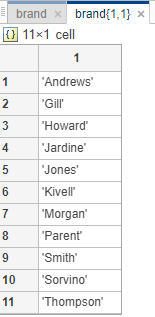Brand stores all the Unique brand that sells the item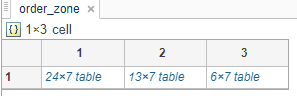Here {1,1} stores central,{1,2} stores east,{1,3} stores west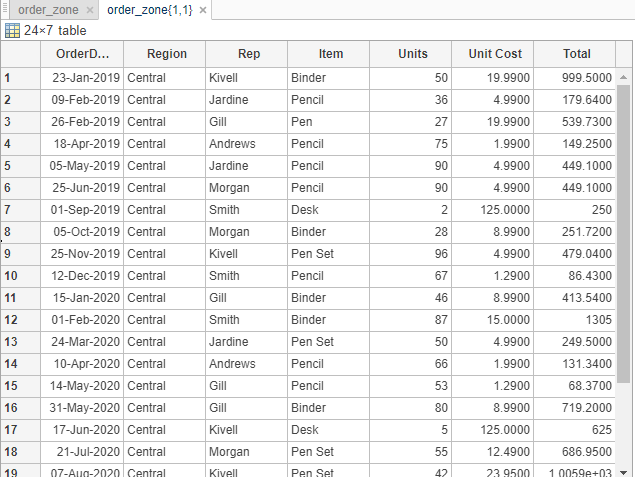Next is Central_order where all the 11 brands with their respective statistics are stored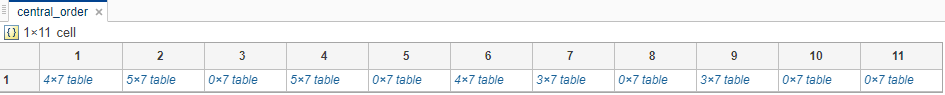The last one is brand_item_C, similar brand_item_E, and brand_item_W is created.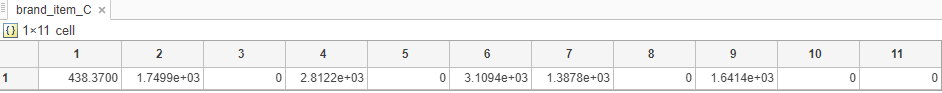Here the total sell for each brand is stored and later sorted to obtain who has the maximum sell. The index having zero is the index where a particular brand does not has any sell.

### Result for determining the best selling brand

The output of the file is as shown.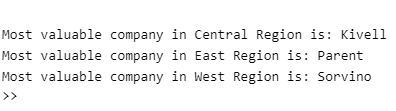The same can be done to calculate the most popular item or maybe many other data.

## Conclusion

Data extracting have multiple-use, one of them being used in Predictive data analysis and the world of Machine Learning. The SWOT analysis of employees in corporate, Engineering analysis data is appropriately stored in the database. Still, it is required to analyze the same, MATLAB comes handy and many many more other applications. So, without wasting any more time, jump right in and explore. Always remember, Love what you do, Do what you love! Happy MATLABing.

Ready to take your MATLAB skills to the next level? Look no further! At MATLAB Helper, we've got you covered. From free community support to expert help and training, we've got all the resources you need to become a pro in no time. If you have any questions or queries, don't hesitate to reach out to us. Simply post a comment below or send us an email at [email protected].

And don't forget to connect with us on LinkedIn, Facebook, and Subscribe to our YouTube Channel! We're always sharing helpful tips and updates, so you can stay up-to-date on everything related to MATLAB. Plus, if you spot any bugs or errors on our website, just let us know and we'll make sure to fix it ASAP.

Ready to get started? Book your expert help with Research Assistance plan today and get personalized assistance tailored to your needs. Or, if you're looking for more comprehensive training, join one of our training modules and get hands-on experience with the latest techniques and technologies. The choice is yours – start learning and growing with MATLAB Helper today!

Education is our future. MATLAB is our feature. Happy MATLABing!

#### Aisik De

Tech freak with a zeal to learn and work on technologies. Acquired degree in Mechatronics , specialized in control system and power electronics.

•Abhishek Agrawal says:

Nice explanation for importing data from excel sheet to MATLAB for processing the data.

#### MATLAB Helper ®

Use Website Chat or WhatsApp at +91-8104622179